Download Presentation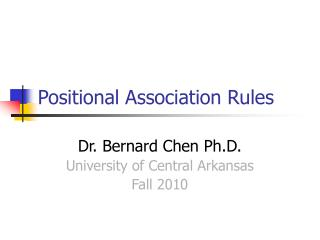Positional Association Rules

# Positional Association Rules - PowerPoint PPT Presentation

Positional Association Rules. Dr. Bernard Chen Ph.D. University of Central Arkansas Fall 2010. Motivation. In order to obtain the DNA/protein sequence motifs information, fixing the length of sequence segments is usually necessary.I am the owner, or an agent authorized to act on behalf of the owner, of the copyrighted work described.
Download Presentation## Positional Association Rules

An Image/Link below is provided (as is) to download presentation

Download Policy: Content on the Website is provided to you AS IS for your information and personal use and may not be sold / licensed / shared on other websites without getting consent from its author.While downloading, if for some reason you are not able to download a presentation, the publisher may have deleted the file from their server.

- - - - - - - - - - - - - - - - - - - - - - - - - - E N D - - - - - - - - - - - - - - - - - - - - - - - - - -
Presentation Transcript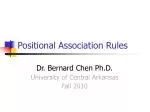### Positional Association Rules

Dr. Bernard Chen Ph.D.

University of Central Arkansas

Fall 2010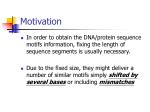Motivation
• In order to obtain the DNA/protein sequence motifs information, fixing the length of sequence segments is usually necessary.
• Due to the fixed size, they might deliver a number of similar motifs simply shifted by several bases or including mismatchesExample
• If there exists a biological sequence motif with length of 12 and we set the window size to 9, it is highly possible that we discovered two similar sequence motifs where one motif covers the front part of the biological sequence motif and the other one covers the rear part.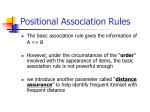Positional Association Rules
• The basic association rule gives the information of

A => B

• However, under the circumstances of the “order” involved with the appearance of items, the basic association rule is not powerful enough
• we introduce another parameter called “distance assurance” to help identify frequent itemset with frequent distance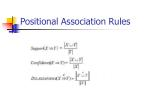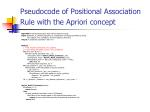Algorithm: Positional Association Rule with the Apriori Concept

Input: Database, D, (Protein sequences as Transactions and Sequence Motifs as items),

min_support, min_confidence, and min_distance_assurance

Output: P, positional association rules in D

Method:

L = find_frequent_itemsets(D, min_support)

S = find_strong_association_rules(L, min_confidence)

for (k=2; Sk ≠ Ø; k++ )

for each strong association rule, r Sk

antecedent_motif = Apriori_Motif_Construct(r_ant)

consequence_motif = Apriori_Motif_Construct(r_con)

if antecident_motif == NULL or consequence_motif == NULL:

goto Step (4)

for each protein sequence, ps D

for (ant_position=1; |ps| ; ant_position++)

if antecedent_motif start appear on ps[ant_position]:

r_ant_count++

for (con_position=1; |ps| ; con_position++)

if consequent_motif start appear on ps[con_position]:

distance = ant_position – con_position

rdistance ++

Pk = { rdistance | rdistance > min_distance_assurance * r_ant_count }

Apriori_Motif_Construct(itemset)

if |itemset| == 1:

return itemset

else:

for each positional association rules in P|itemset|

if all items in the itemset appear in the positional association rule:

return the new motif constructed by the positional association rule

return NULL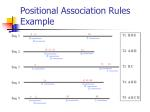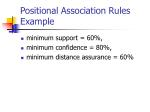Positional Association Rules Example
• minimum support = 60%,
• minimum confidence = 80%,
• minimum distance assurance = 60%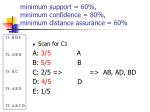minimum support = 60%, minimum confidence = 80%, minimum distance assurance = 60%
• Scan for C1

A: 3/5 A

B: 5/5 B

C: 2/5 => => AB, AD, BD

D: 4/5 D

E: 1/5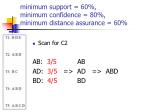minimum support = 60%, minimum confidence = 80%, minimum distance assurance = 60%
• Scan for C2

AB: 3/5 AB

BD: 4/5BDminimum support = 60%, minimum confidence = 80%, minimum distance assurance = 60%
• Scan for C3

ABD: 3/5 => ABD => no C4minimum support = 60%, minimum confidence = 80%, minimum distance assurance = 60%
• Therefore, the itemset that pass support: {AB, AD, BD, ABD}
• Next, we need to compute their confidenceminimum support = 60%, minimum confidence = 80%, minimum distance assurance = 60%
• First, we work on 2-itemset:

A=>B: 3/3

B=>A: 3/5

A=>D: 3/3

D=>A: 3/4

B=>D: 4/5

D=>B: 4/4minimum support = 60%, minimum confidence = 80%, minimum distance assurance = 60%
• then, we work on 3-itemset:

{ABD}

A=>BD: 3/3

D=>AB: 3/4

AB=>D: 3/3

BD=>A: 3/4minimum support = 60%, minimum confidence = 80%, minimum distance assurance = 60%
• Thus, the strong association rules we have:

2-itemset 3-itemset

A=>B A=>BD

A=>D AB=>D

D=>B

Next, we work on Positional Association rules…1.= 3/4 3. =1/4

2. = 1/41.= 3/6 3. = 1/6

2. = 1/61.= 2/4 3. = 1/4

2. = 1/4 4. = 1/41.= 3/4

2. = 1/41.= 2/3

2. = 1/3NO Positional Association Rules on AB !!!1.= 2/4

2. = 1/4### Strong Association Rules are not necessary interesting

Dr. Bernard Chen Ph.D.

University of Central Arkansas

Fall 2010Example 5.8 Misleading “Strong” Association Rule
• Of the 10,000 transactions analyzed, the data show that
• 6,000 of the customer included computer games,
• while 7,500 include videos,
• And 4,000 included both computer games and videosMisleading “Strong” Association Rule
• For this example:
• Support (Game & Video) =

4,000 / 10,000 =40%

• Confidence (Game => Video) =

4,000 / 6,000 = 66%

• Suppose it pass our minimum support and confidence (30% , 60%, respectively)Misleading “Strong” Association Rule
• However, the truth is : “computer games and videos are negatively associated”
• Which means the purchase of one of these items actually decreases the likelihood of purchasing the other.
• (How to get this conclusion??)Misleading “Strong” Association Rule
• Under the normal situation,
• 60% of customers buy the game
• 75% of customers buy the video
• Therefore, it should have 60% * 75% = 45% of people buy both
• That equals to 4,500 which is more than 4,000 (the actual value)From Association Analysis to Correlation Analysis
• Lift is a simple correlation measure that is given as follows
• The occurrence of itemset A is independent of the occurrence of itemset B if

P(AUB) = P(A)P(B)

• Otherwise, itemset A and B are dependent and correlated as events
• Lift(A,B) = P(AUB) / P(A)P(B)
• If the value is less than 1, the occurrence of A is negatively correlated with the occurrence of B
• If the value is greater than 1, then A and B are positively correlated